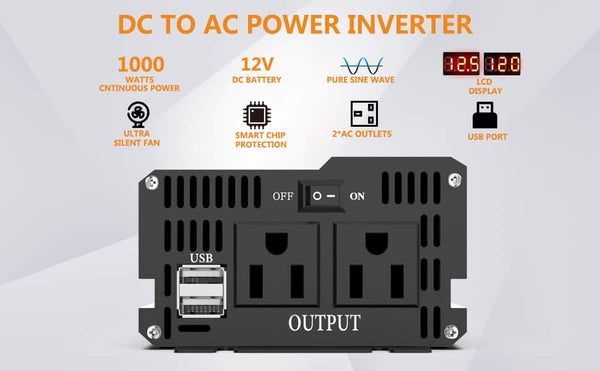# How an Inverter Converts 12V DC to 120V AC

Inverters are used to convert DC power to AC power. As a power converter device, it plays a vital role in many situations where power cannot be obtained from the mains.
For example, to turn 12V DC into 120V AC. the inverter converts DC power (from a battery) into constant frequency, constant voltage, or FM AC power (usually 120V/50Hz starting wave). It consists of an inverter bridge, control logic, and filter circuitry. By adding additional MOSFET transistors, it can also drive more powerful loads. Thus, with a complete set of inverters, you can input low voltage DC and output 120V AC.## What is the difference between 12V DC and 120V AC

There are a few key differences between 12V DC and 120V AC power. For one, 12V DC is the direct current while 120V AC is the alternating current. This means that the electricity flows in one direction with DC power, while it alternates back and forth with AC power. AC power is the type of power that comes from your outlets at home, while DC power is often used in car batteries and solar panels.

Another difference between these two types of power is the voltage. 12V DC power has a lower voltage than 120V AC power. This means that it is not as powerful and can't be used for things that require a lot of power, like appliances. DC power is often used for things like small electronics and LED lights.

So, when it comes to 12V DC vs 120V AC, the main differences are the type of current, the voltage, and the power. AC power is the type of power that you use at home, while DC power is often used in car batteries and solar panels.

## How do you convert 12V DC (Direct Current) to 120V AC (Alternating Current)

The inverter converts the AC voltage from the grid/PV to a stable 12V DC output, and the inverter converts the 12V DC voltage output from the adapter to high-frequency high-voltage AC 120V
12V DC → high-frequency boost → 120V DC → full bridge rectifier → 120V DC → inverter bridge rectifier → 120V AC

In addition, the inverter itself will consume some power when working, so its input power must be greater than its output power. The efficiency of an inverter is the ratio of the output power to the input power. Let's say an inverter has an input of 100 watts DC and an output of 90 watts AC, then its efficiency is 90%.## What are the benefits of using an inverter

An inverter is a device that converts DC (direct current) into AC (alternating current). DC is the kind of electricity that comes from batteries, while AC is the kind of electricity that comes from the power grid.

There are several benefits of using a pure sine wave inverter.

First, pure sine wave inverters produce clean and stable AC power. This is important because many devices, such as computers and sensitive electronic equipment, require clean and stable power to function properly.

Second, pure sine wave inverters are more efficient than other types of inverters. This means that they use less energy to produce the same amount of AC power. This can save you money on your energy bill.For more information about Types of Inverters, you can check this article: Types of Inverters.

Third, pure sine wave inverters create less electromagnetic interference than other types of inverters. This is important because electromagnetic interference can cause problems with electronic equipment.

Fourth, pure sine wave inverters are less likely to damage electronic equipment. This is because the waveform produced by a pure sine wave inverter is less likely to damage electronic components.

Finally, pure sine wave inverters are typically more expensive than other types of inverters. However, the benefits of using a pure sine wave inverter may outweigh the cost for some people.k output power?